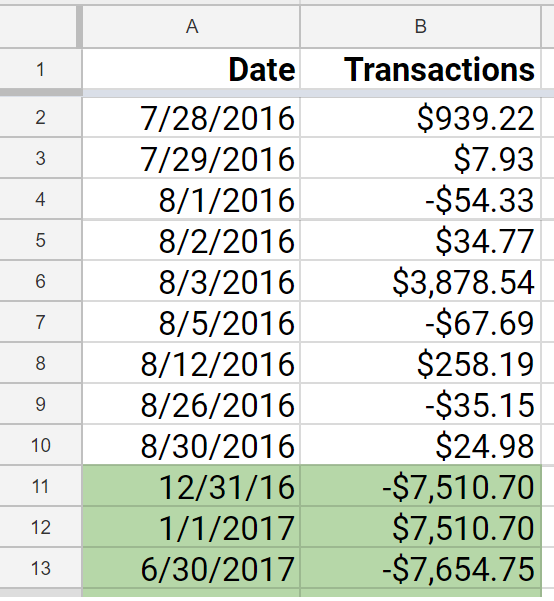Friday, 30 June 2017 06:41

How to calculate your internal rate of return using Google Sheets

Written by

The internal rate of return (IRR) is the dollar-weighted return of a particular investment. It is what I consider to be gold standard measurement of your investment's personal performance. The calculation for IRR looks like a pain in the ass. Luckily, there is a spreadsheet function that calculates this easily for you, and I just so happen to be awesome at creating spreadsheets.I use Google Sheets to automatically calculate my internal rate of return (IRR) for all of my investment accounts and to create my performance chart.  While it is not really necessary to track your IRR so long as you are following your target asset allocation, I still enjoy knowing that I am tracking along as planned.  The spreadsheet I made also happens to calculate my asset allocation on a realtime basis, but that will be too complicated to teach in a post.  In this post, I will teach you how to calculate the IRR with Google Sheets.  The same function also works for Excel, but I do like that I can access Sheets from anywhere easily.

Information to Gather Before Calculating the IRR

Here is the information you need before you can calculate your IRR

• The balance of your investment(s) at the start of the time period of interest
• All contributions and withdrawals (dates and amounts) you have made during this period
• The ending balance

Below is an example using my Robinhood Brokerage Account, which I started just to play with picking stocks. By the way, I do not recommend this as an investment strategy.  This account is purely for fun and speculation.I have listed the dates of all transactions and the transaction amount side by side.  A positive amount indicates that I put money into a stock.  A negative amount means that I sold stocks. Some of these amounts are small because they represent the net total for that day after exchanging one stock for another. If you earned a dividend and did not reinvest it (i.e. you withdrew it from the account), then you should add a negative value transaction to your list.  If you don't do this, then your calculated IRR will be lower than the actual value.

I calculate my IRR on a yearly basis, so my desired end date is 12/31/16 in this case. Most of your investment accounts will give you quarterly and annual summaries, which will conveniently give you your balances at that time. My balance at the end of the year was \$7,510.70.

On 12/31/16, you can see that I put -\$7,510.70. The reason this is a negative value is because you have to pretend to sell the stock in order to determine its return.  This is just to make the function work.  The next day, on 1/1/17, I add back the same amount. The last row is a formula to specify today's date, and the value is the negative realtime account balance using the GOOGLEFINANCE function.  Once again, it is negative because you need to pretend to sell the stock to calculate the return.

The XIRR Function

XIRR(cashflow_amounts, cashflow_dates, [rate_guess])

Above is the formula that you use to call the XIRR function. In parentheses are the parameters you need to provide to the XIRR function.  The last parameter is in square brackets, meaning it is optional. Here is a description of what the first two variables mean:

• cashflow_amounts - all of the transaction amounts corresponding to your time period of interest
• cashflow_dates - the dates of all transactions specified in the same order

Below is an example of the XIRR function and how I used it to calculate my IRR.On the far right you can examine the formula that calculates your IRR for those time periods.  And Yes! I did have an IRR of 169.6% on this account in 2016 (The stock increased by 40% in the short time that I owned it). Individual stocks are volatile like that! I am keeping it for at least a year though so I can sell it at long term capital gains tax rate.